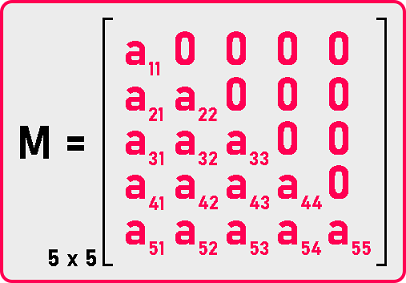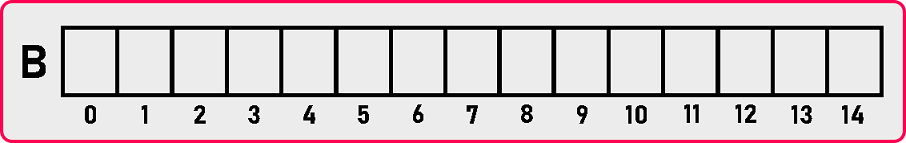# Lower Triangular Matrix Column Major Mapping

## Lower Triangular Matrix by Column Major Mapping in C and C++:

In this article, I am going to discuss Lower Triangular Matrix by Column Major Mapping in C and C++ Language with Examples. Please read our previous article, where we discussed Lower Triangular Matrix by Row-Major Mapping in C and C++ Language with Examples.

##### Lower Triangular Matrix by Column Major Mapping:

Now let us look at the column-major formula and store the elements column by column in a single dimension. This is the matrix that we want to store in a single dimension array.We have to create an array of size 15 or we can calculate the size of the array by using the below formula: = n * (n +1) / 2.Here we have created an array of size 15. Now we have to insert elements of the matrix by column-major mapping.

###### 1st Column:###### 2nd Column:###### 3rd Column:###### 4th Column:###### 5th Column:So, we have filled elements column by column but there must be some formula to map these elements from a two-dimensional matrix to a single dimension array. For that, we will take some examples and we will do some observation and then we will prepare the formula for it. Let us take an example M :M  is present on the 12th index in the array. So, we have to find Index (M ).We have to reach on 4th column to find the target element. So, we need to skip 3 columns to reach the 4th column.So, 1st column having 5 elements: Index (M ) = 5 +

2nd column having 4 elements: Index (M ) = 5 + 4 +

3rd column having 3 elements: Index (M ) = 5 + 4 + 3

We have skipped 3 columns ‘1’, ‘2’ and ‘3’.

Now we are pointing at the beginning of the 4th column. Do we have to move ahead to get on the element? No, we are on the element. So,

Index (M ) = [5 + 4 + 3] + 0

Index (M ) = 12

We got 12 here. So, this is true. Next, we want the element M  which is in the same column as the previous element M . Let us get the index for M . This is again in the fourth column.

For reaching the 4th column, again we have to skip 3 columns ‘1’, ‘2’ and ‘3’. So, the expression will be: Index (M ) = 5 + 4 + 3

Now how much we should move ahead? Just one element we should move forward. So, add 1 to the above expression.

Index (M ) = [5 + 4 + 3] + 1

How this can be converted into the formula for any index? By observing previous example, for any Index (M [i][j]) formula will be:

Index (M [i][j]) = [n * (j – 1) – (j – 2)(j – 1)/2] + (i – j)

Where n is the dimension of the matrix, i is the row number, and j is the column number. Now let’s look at the code part:

##### Lower Triangular Matrix by Column Major Mapping Code in C Language:
```#include <stdio.h>
#include <stdlib.h>

struct Matrix
{
int *B;
int n;
};

void Set(struct Matrix *m, int i, int j, int y)
{
if (i >= j)
m->B[(m->n * (j - 1) - (j - 2) * (j - 1) / 2) + (i - j)] = y;
}

int Get(struct Matrix m, int i, int j)
{
if (i >= j)
return m.B[(m.n * (j - 1) - (j - 2) * (j - 1) / 2) + (i - j)];
else
return 0;
}

void Display(struct Matrix m)
{
int i, j;
printf ("\nMatrix is: \n");
for (i = 1; i <= m.n; i++)
{
for (j = 1; j <= m.n; j++)
{
if (i >= j)
printf ("%d ", m.B[(m.n * (j - 1) - (j - 2) * (j - 1) / 2) + (i - j)]);
else
printf ("0 ");
}
printf ("\n");
}
}

int main()
{
struct Matrix M;
int i, j, y;

printf("Enter Dimension of Matrix: ");
scanf("%d", &M.n);
M.B = (int *) malloc (M.n * (M.n + 1) / 2 * sizeof(int));

printf("Enter all the elements of the matrix:\n");
for (i = 1; i <= M.n; i++)
{
for(j = 1; j <= M.n; j++)
{
scanf("%d", &y);
Set(&M, i, j, y);
}
}

Display(M);
}
```
###### Output:##### Lower Triangular Matrix by Column Major Mapping Code in C++ Language:
```#include <iostream>
using namespace std;

class LowerTriangularMatrix
{
private:
int *B;
int n;

public:
LowerTriangularMatrix ()
{
n = 3;
B = new int[3 * (3 + 1) / 2];
}

LowerTriangularMatrix (int n)
{
this->n = n;
B = new int[n * (n + 1) / 2];
}

~LowerTriangularMatrix ()
{
delete[]B;
}

int GetDimension ()
{
return n;
}

void Set (int i, int j, int y);
int Get (int i, int j);
void Display ();
};

void LowerTriangularMatrix::Set (int i, int j, int y)
{
if (i >= j)
B[(n * (j - 1) - (j - 2) * (j - 1) / 2) + (i - j)] = y;
}

int LowerTriangularMatrix::Get (int i, int j)
{
if (i >= j)
return B[(n * (j - 1) - (j - 2) * (j - 1) / 2) + (i - j)];
return 0;
}

void LowerTriangularMatrix::Display ()
{
cout << "\nMatrix is: " << endl;
for (int i = 1; i <= n; i++)
{
for (int j = 1; j <= n; j++)
{
if (i >= j)
cout << B[(n * (j - 1) - (j - 2) * (j - 1) / 2) + (i - j)] << " ";
else
cout << "0 ";
}
cout << endl;
}
}

int main ()
{
int d;
cout << "Enter Dimensions: ";
cin >> d;

LowerTriangularMatrix lm (d);

int x;
cout << "Enter All Elements: " << endl;
for (int i = 1; i <= d; i++)
{
for (int j = 1; j <= d; j++)
{
cin >> x;
lm.Set (i, j, x);
}
}

lm.Display ();
return 0;
}
```
###### Output:In the next article, I am going to discuss Upper Triangular Matrix Row Major Mapping in C and C++ Language with Examples. Here, in this article, I try to explain Lower Triangular Matrix by Column Major Mapping in C and C++ Language with Examples and I hope you enjoy this Lower Triangular Matrix by Column Major Mapping in C and C++ with Examples article.